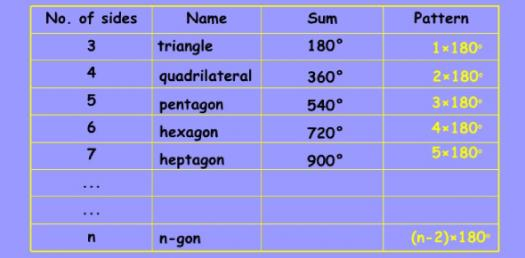# Properties Of Polygons

14 Questions | Total Attempts: 518SettingsThis quiz is created for sixth grade math. The students are required to master 2-D shapes and thier properties.

Related Topics
• 1.
A shape that contains only one pair of pararellel sides.
• A.

Rhombus

• B.

Trapezoid

• C.

Square

• D.

Triangle

• 2.
A shape that has four equal sides and opposite angles equal.
• A.

Square

• B.

Rhombus

• C.

Both a and b

• D.

Neither a or b

• 3.
A shape that has four congruent sides and four right angles.
• A.

Square

• B.

Rectangle

• C.

Rhombus

• D.

Trapezoid

• 4.
A shape that has one right angle.
• A.

Acute triangle

• B.

Obtuse triangle

• C.

Equilateral triangle

• D.

Right triangle

• 5.
All squares are rectangles.
• A.

True

• B.

False

• 6.
All quadrilaterals have one pair of parallel sides.
• A.

True

• B.

False

• 7.
All rectangles are squares.
• A.

True

• B.

False

• 8.
A triangles with all three sides equal is called _____________.
• 9.
A triangle with only two sides equal is called an ________________.
• 10.
The sum of the angles in a triangle is ___________.
• 11.
The sum of the four angles in a quadrilateral is ______________.
• 12.
Each angle in an equilateral triangles measures _____________.
• 13.
All squares are a rhombus.  Explain why this statement is true or false.
• 14.
Can you make a triangle with sides measuring 5 inches, 7 inches, and 12 inches?  Why or why not?